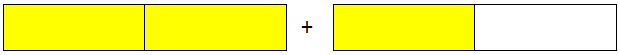### Fractions

Proper fraction:

Fractions whose numerators are less than the denominators are called proper fractions. (Numerator < denominator)

For examples:

, etc are proper fractions.

Two parts are shaded in the above diagram. The total number of equal parts is 3. Therefore, the shaded part can be represented as an infraction. The numerator (top number) is less compared to the denominator (bottom number). This type of fraction is called a proper fraction.

Similarly,

Three parts are shaded in the above diagram. The total number of equal parts is 4. Therefore, the shaded part can be represented as infractions. The numerator (top number) is less compared to the denominator (bottom number). This type of fraction is called a proper fraction.

Note: The value of a proper fraction is always less than 1.

Improper fraction:

Fractions with the numerator either equal to or greater than the denominator are called an improper fractions. (Numerator = denominator or, Numerator > denominator)

Fractions like etc. are not proper fractions. These are improper fractions. The fraction is an improper fraction.

The fractions are examples of improper fractions. The top number (numerator) is greater than the bottom number (denominator). Such type of fraction is called an improper fraction.

Notes:

(i) Every natural number can be written as a fraction in which 1 is its the denominator. For example, 21., = 25 =, 53 =, etc. So every natural number is an improper fraction.

(ii) The value of an improper fraction is always equal to or greater than 1.

Mixed fraction:

A combination of a proper fraction and a whole number is called a mixed fraction.Now, you have three half or you can say that you have a whole and a half or .

A number such as is a mixed number.

In other words:

A fraction that contains two parts: (i) a natural number and (ii) a proper fraction, is called a mixed fraction, e.g., 3, etc.

Note: A mixed number is formed with a whole number and a fraction.

Property 1:

A mixed fraction may always be converted into an improper fraction.

Multiply the natural number by the denominator and add to the numerator. This new numerator over the denominator is the required fraction.

Property 2:

An important fraction can be always be converted into a mixed fraction.

Divide the numerator by the denominator to get the quotient and remainder. Then the quotient is the natural number part and the remainder over the denominator is the proper fraction part of the required mixed fraction.

Example: can be converted into a mixed fraction as follows:

7
6 |43
– 42
1

Dividing 43 by 6, we get quotient = 7 and remainder = 1.

Note: Proper fraction is between 0 to 1. An improper fraction is 1 or greater than 1. A mixed fraction is greater than 1.

1. Write as a mixed fraction.

Solution:

So, Quotient = 9, Remainder = 1 and Divisor = 4

2. Classify the following as proper fractions, improper fractions or unit fractions.